# 24 Multiplexing_Techniques.pdf

28. May 2023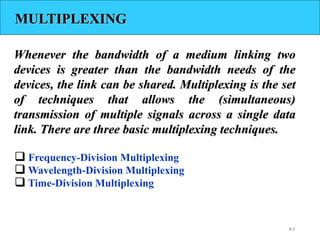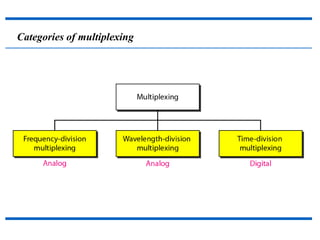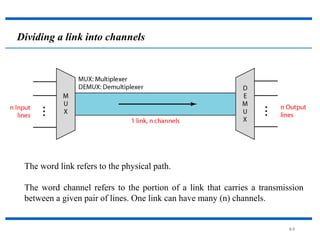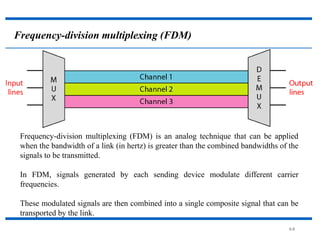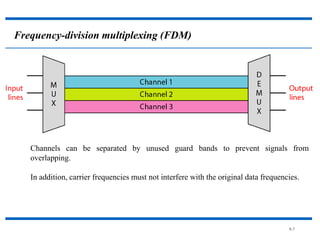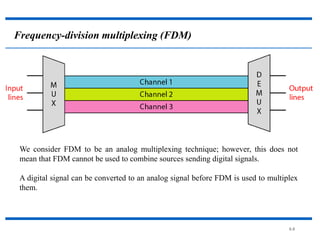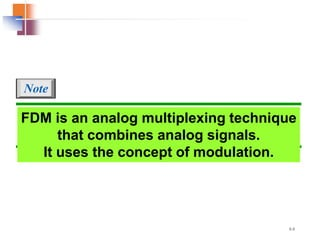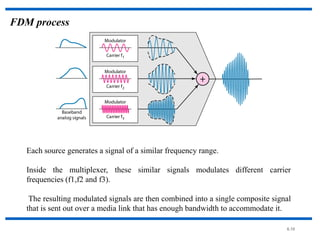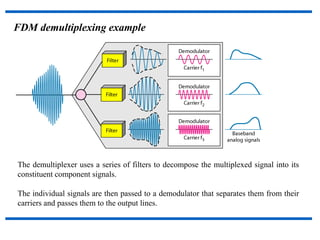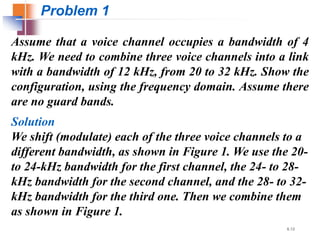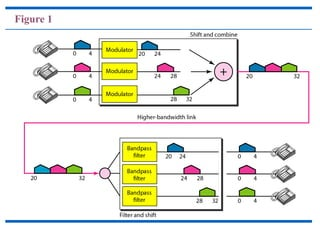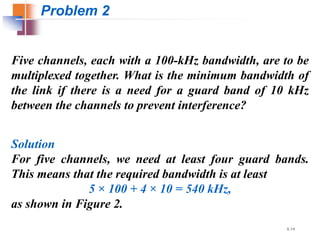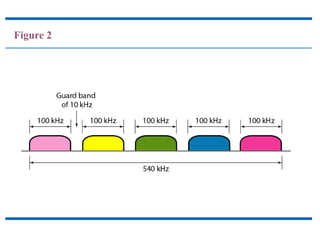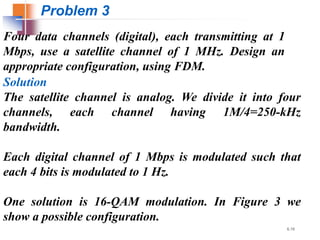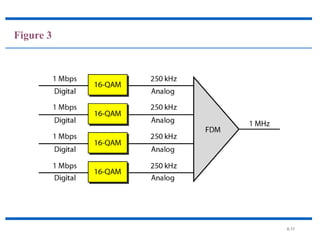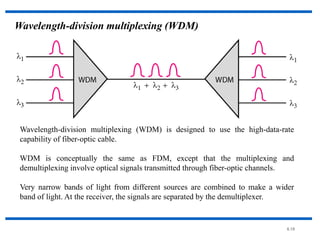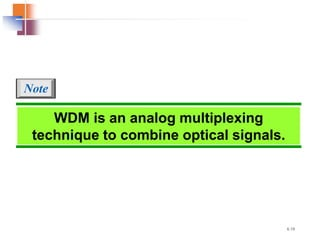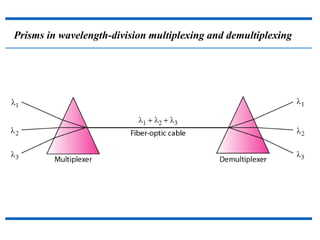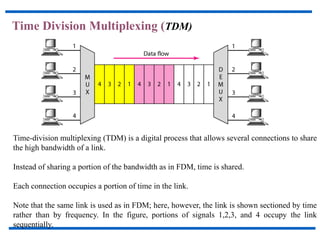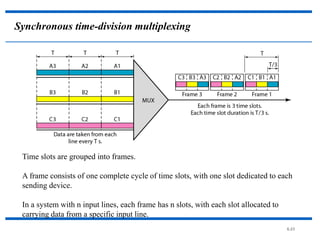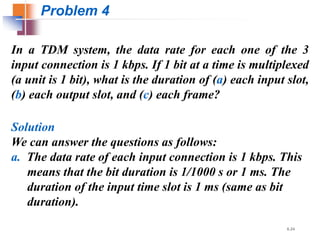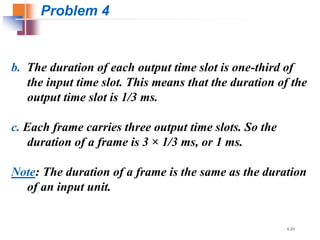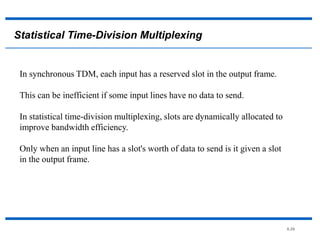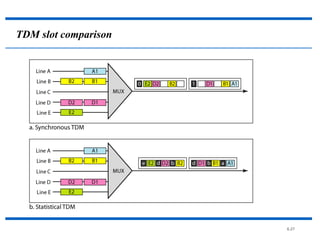1 von 27

### 24 Multiplexing_Techniques.pdf

• 1. Multiplexing Techniques Dr. G.Aarthi, Associate Professor, School of Electronics Engineering
• 2. 6.2 Bandwidth utilization is the wise use of available bandwidth to achieve specific goals. Efficiency can be achieved by multiplexing; i.e., sharing of the bandwidth between multiple users. Note
• 3. 6.3 MULTIPLEXING Whenever the bandwidth of a medium linking two devices is greater than the bandwidth needs of the devices, the link can be shared. Multiplexing is the set of techniques that allows the (simultaneous) transmission of multiple signals across a single data link. There are three basic multiplexing techniques.  Frequency-Division Multiplexing  Wavelength-Division Multiplexing  Time-Division Multiplexing
• 4. Categories of multiplexing
• 5. 6.5 Dividing a link into channels The word link refers to the physical path. The word channel refers to the portion of a link that carries a transmission between a given pair of lines. One link can have many (n) channels.
• 6. 6.6 Frequency-division multiplexing (FDM) Frequency-division multiplexing (FDM) is an analog technique that can be applied when the bandwidth of a link (in hertz) is greater than the combined bandwidths of the signals to be transmitted. In FDM, signals generated by each sending device modulate different carrier frequencies. These modulated signals are then combined into a single composite signal that can be transported by the link.
• 7. 6.7 Frequency-division multiplexing (FDM) Channels can be separated by unused guard bands to prevent signals from overlapping. In addition, carrier frequencies must not interfere with the original data frequencies.
• 8. 6.8 Frequency-division multiplexing (FDM) We consider FDM to be an analog multiplexing technique; however, this does not mean that FDM cannot be used to combine sources sending digital signals. A digital signal can be converted to an analog signal before FDM is used to multiplex them.
• 9. 6.9 FDM is an analog multiplexing technique that combines analog signals. It uses the concept of modulation. Note
• 10. 6.10 FDM process Each source generates a signal of a similar frequency range. Inside the multiplexer, these similar signals modulates different carrier frequencies (f1,f2 and f3). The resulting modulated signals are then combined into a single composite signal that is sent out over a media link that has enough bandwidth to accommodate it.
• 11. FDM demultiplexing example The demultiplexer uses a series of filters to decompose the multiplexed signal into its constituent component signals. The individual signals are then passed to a demodulator that separates them from their carriers and passes them to the output lines.
• 12. 6.12 Assume that a voice channel occupies a bandwidth of 4 kHz. We need to combine three voice channels into a link with a bandwidth of 12 kHz, from 20 to 32 kHz. Show the configuration, using the frequency domain. Assume there are no guard bands. Solution We shift (modulate) each of the three voice channels to a different bandwidth, as shown in Figure 1. We use the 20- to 24-kHz bandwidth for the first channel, the 24- to 28- kHz bandwidth for the second channel, and the 28- to 32- kHz bandwidth for the third one. Then we combine them as shown in Figure 1. Problem 1
• 13. Figure 1
• 14. 6.14 Five channels, each with a 100-kHz bandwidth, are to be multiplexed together. What is the minimum bandwidth of the link if there is a need for a guard band of 10 kHz between the channels to prevent interference? Solution For five channels, we need at least four guard bands. This means that the required bandwidth is at least 5 × 100 + 4 × 10 = 540 kHz, as shown in Figure 2. Problem 2
• 15. Figure 2
• 16. 6.16 Four data channels (digital), each transmitting at 1 Mbps, use a satellite channel of 1 MHz. Design an appropriate configuration, using FDM. Solution The satellite channel is analog. We divide it into four channels, each channel having 1M/4=250-kHz bandwidth. Each digital channel of 1 Mbps is modulated such that each 4 bits is modulated to 1 Hz. One solution is 16-QAM modulation. In Figure 3 we show a possible configuration. Problem 3
• 17. 6.17 Figure 3
• 18. 6.18 Wavelength-division multiplexing (WDM) Wavelength-division multiplexing (WDM) is designed to use the high-data-rate capability of fiber-optic cable. WDM is conceptually the same as FDM, except that the multiplexing and demultiplexing involve optical signals transmitted through fiber-optic channels. Very narrow bands of light from different sources are combined to make a wider band of light. At the receiver, the signals are separated by the demultiplexer.
• 19. 6.19 WDM is an analog multiplexing technique to combine optical signals. Note
• 20. Prisms in wavelength-division multiplexing and demultiplexing
• 21. Time Division Multiplexing (TDM) Time-division multiplexing (TDM) is a digital process that allows several connections to share the high bandwidth of a link. Instead of sharing a portion of the bandwidth as in FDM, time is shared. Each connection occupies a portion of time in the link. Note that the same link is used as in FDM; here, however, the link is shown sectioned by time rather than by frequency. In the figure, portions of signals 1,2,3, and 4 occupy the link sequentially.
• 22. 6.22 TDM is a digital multiplexing technique for combining several low-rate digital channels into one high-rate one. Note
• 23. 6.23 Synchronous time-division multiplexing Time slots are grouped into frames. A frame consists of one complete cycle of time slots, with one slot dedicated to each sending device. In a system with n input lines, each frame has n slots, with each slot allocated to carrying data from a specific input line.
• 24. 6.24 In a TDM system, the data rate for each one of the 3 input connection is 1 kbps. If 1 bit at a time is multiplexed (a unit is 1 bit), what is the duration of (a) each input slot, (b) each output slot, and (c) each frame? Solution We can answer the questions as follows: a. The data rate of each input connection is 1 kbps. This means that the bit duration is 1/1000 s or 1 ms. The duration of the input time slot is 1 ms (same as bit duration). Problem 4
• 25. 6.25 b. The duration of each output time slot is one-third of the input time slot. This means that the duration of the output time slot is 1/3 ms. c. Each frame carries three output time slots. So the duration of a frame is 3 × 1/3 ms, or 1 ms. Note: The duration of a frame is the same as the duration of an input unit. Problem 4
• 26. 6.26 Statistical Time-Division Multiplexing In synchronous TDM, each input has a reserved slot in the output frame. This can be inefficient if some input lines have no data to send. In statistical time-division multiplexing, slots are dynamically allocated to improve bandwidth efficiency. Only when an input line has a slot's worth of data to send is it given a slot in the output frame.
• 27. 6.27 TDM slot comparison